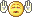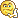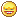We are here with you hands in hands to facilitate your learning & don't appreciate the idea of copying or replicating solutions. Read More>>

#www.vustudents.ning.com

 www.bit.ly/vucodes + Link For Assignments, GDBs & Online Quizzes Solution www.bit.ly/papersvu + Link For Past Papers, Solved MCQs, Short Notes & More

Dear Students! Share your Assignments / GDBs / Quizzes files as you receive in your LMS, So it can be discussed/solved timely. Add Discussion

Assignment No.3 (Course STA301)
Fall 2012 (Total Marks 15)
Your Assignment must be uploaded/ submitted before or on 23:59 January 16th, 2013
STUDENTS ARE STRICTLY DIRECTED TO SUBMIT THEIR ASSIGNMENT BEFORE OR BY DUE DATE. NO ASSIGNMNENT AFTER DUE DATE WILL BE ACCEPTED VIA E.MAIL).
Rules for Marking
It should be clear that your Assignment will not get any credit IF:
 The Assignment submitted, via email, after due date.
 The submitted Assignment is not found as MS Word document file.
 There will be unnecessary, extra or irrelevant material.  The Statistical notations/symbols are not well-written i.e., without using MathType software.
 The Assignment will be copied from handouts, internet or from any other student’s file. Copied material (from handouts, any book or by any website) will be awarded ZERO MARKS. It is PLAGIARISM and an Academic Crime.
 The medium of the course is English. Assignment in Urdu or Roman languages will not be accepted.
 Assignment means Comprehensive yet precise accurate details about the given topic quoting different sources (books/articles/websites etc.). Do not rely only on handouts. You can take data/information from different authentic sources (like books, magazines, website etc) BUT express/organize all the collected material in YOUR OWN WORDS. Only then you will get good marks.
Objective(s) of this Assignment:
 Properties of probability distributions.
 Application of probability distribution in real life.
 Mathematical expectation and its properties.
Assignment No: 3 (Lessons 23-30)
Question 1: Marks: 4+4=8
a) Given the function below
f (x)  c(1 x) 0  x 1
Obtain the value of “c”, so that f (x) is a density function.
b) A random variable “X” following binomial distribution has its mean and variance,
18 and 3.52 respectively. Calculate the value of “n” and “p”.
Question 2: Marks: 5+2=7
a) During a laboratory experiment the number of radio active particles passing
through a counter in one milli-second is 4.
I. What is the probability that 6 particles enter the counter in a given millisecond?
II. What is the probability that at most two particles enter the counter in a given
milli-second?
b) If E(X)  5 , then calculate the value of E(Y) . Where Y is defined as
Y  3X 5
-END

+ How to Join Subject Study Groups & Get Helping Material?

+ How to become Top Reputation, Angels, Intellectual, Featured Members & Moderators?

+ VU Students Reserves The Right to Delete Your Profile, If?

Views: 13663

.

+ http://bit.ly/vucodes (Link for Assignments, GDBs & Online Quizzes Solution)

+ http://bit.ly/papersvu (Link for Past Papers, Solved MCQs, Short Notes & More)

Attachments:

### Replies to This Discussion

Our main purpose here discussion not just Solution

All students must share points and also your problems.... so it can discussed and solution can prepare..

Dont wait for solution just participate in Discussions becoz after discussions a correct solution will prepare...

yaar koi kuch tu help kro hanout mai refrence hi de do..

Q1 b)

b) A random variable “X” following binomial distribution has its mean and variance, 18 and 3.52 respectively. Calculate the value of “n” and “p”.

solution

mean=np=18

variance=npq=3.52

npq/np=0.195=q

p=1-q

=1-.195=.80

np=18

n(.80)=18

n=22.50thnax Anaya but can u give reference k ap ne kaha se solve kia hai?book ma dkho sara formula hamere pass sirf handout hn but i get it in handout onething i don't get that p=1-q

E(X)=5 then E(Y)=?

Y=3X+5

Y=3*5+5=20

or

Y=20

what is differenec bt Y and E(Y) ??then how to get E(Y)p+q = 1 ya to ho ga formula is ko p=1-q kya ha

thnx

If a discrete random variable X has the following probability density function (p.d.f.), it is said to have a binomial distribution:

• P(X = x) = nCx q(n-x)px, where q = 1 - p

p can be considered as the probability of a success, and q the probability of a failure.

so plzz frnds keep in mind that don't use short cut in life in every field,, is mn ap ka hi faida heher kam thru proper channel kro, thanx 4 ur co-operation.

q.1 part b ka answer share karien....and tell me is there any m.com student???

.